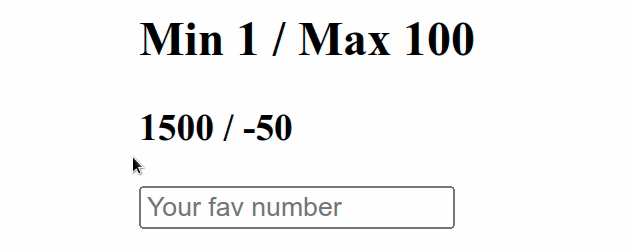# Create numeric input with Min and Max validation in ReactLast updated: Jan 15, 2023
2 min## #Create numeric input with Min and Max validation in React

To create a numeric input with min and max validation in React:

1. Define an input with `type` set to `number`.
2. Set an `onChange` event handler on the input field.
3. Every time the value changes assign a number from min to max to a state variable.App.js
```Copied!```import {useState} from 'react';

const App = () => {
const min = 1;
const max = 100;

const [value, setValue] = useState(1);

const handleChange = event => {
const value = Math.max(min, Math.min(max, Number(event.target.value)));
setValue(value);
};

console.log(value);
console.log(typeof value);

return (
<div>
<h2>Min 1 / Max 100</h2>
<h3>1500 / -50</h3>
<input
type="number"
value={value}
onChange={handleChange}
/>
</div>
);
};

export default App;
``````

We used the useState hook to keep the value in the component's state.

Every time the value of the input type `number` changes, we use the Math.max function to get a number from `min` up to `max`.

The `Math.max` function takes zero or more numbers and returns the largest of the given numbers.

We used the Math.min function to get a number between the predefined `max` and what the user typed in.

The `Math.min` function returns the lowest value from the zero or more provided numbers.

The example above only allows for numbers between `1` and `100`.

If the user tries to type in a number lower than `1`, we simply set the predefined `min` value to the state variable.

On the other hand, if the user tries to type in a larger value than the `max`, we set the state variable to the predefined `max`.

In all other cases, the state variable is set to the number the user provided.

I've also written an article on how to create a numbers-only input field.

I wrote a book in which I share everything I know about how to become a better, more efficient programmer.You can use the search field on my Home Page to filter through all of my articles.